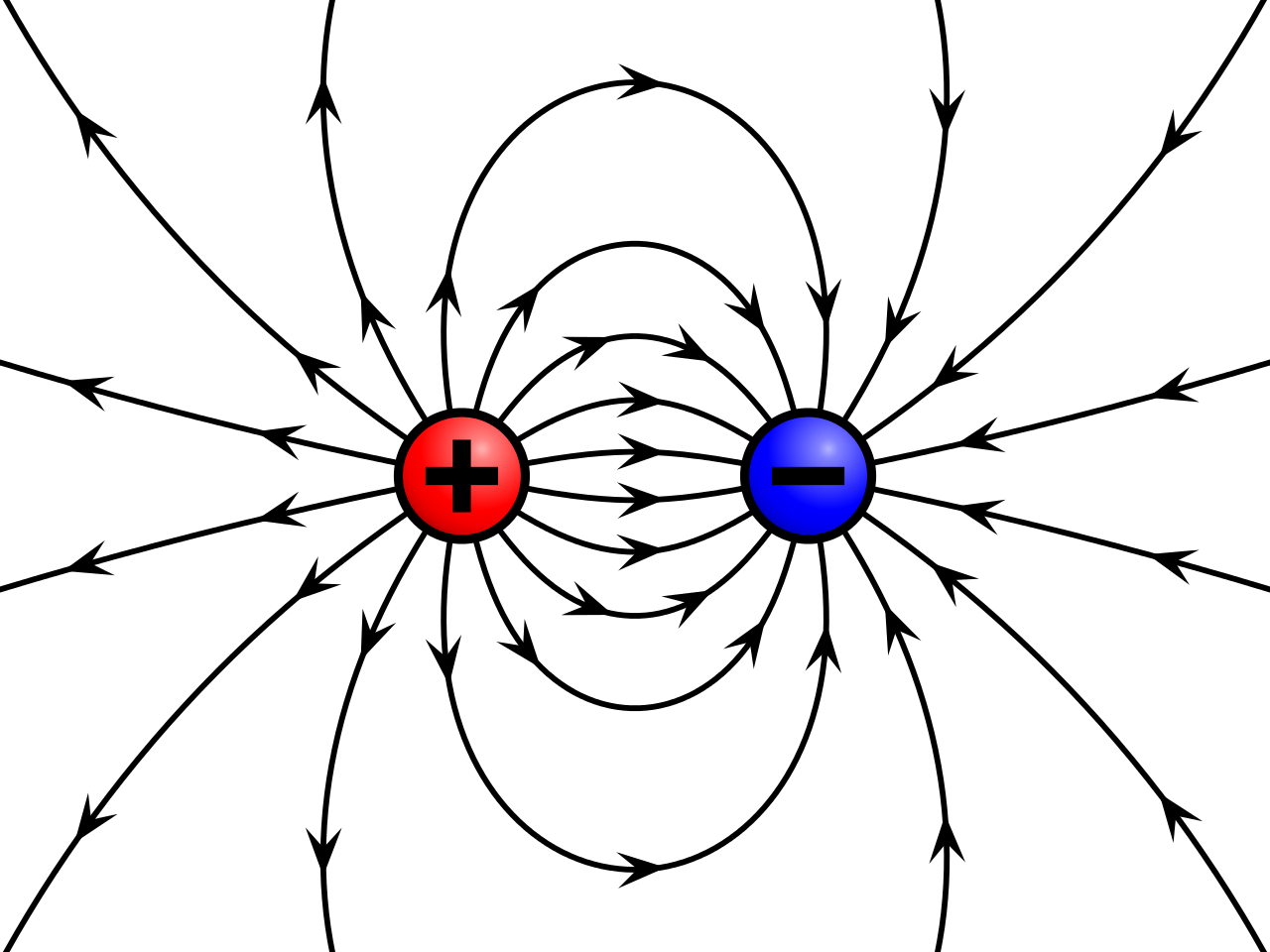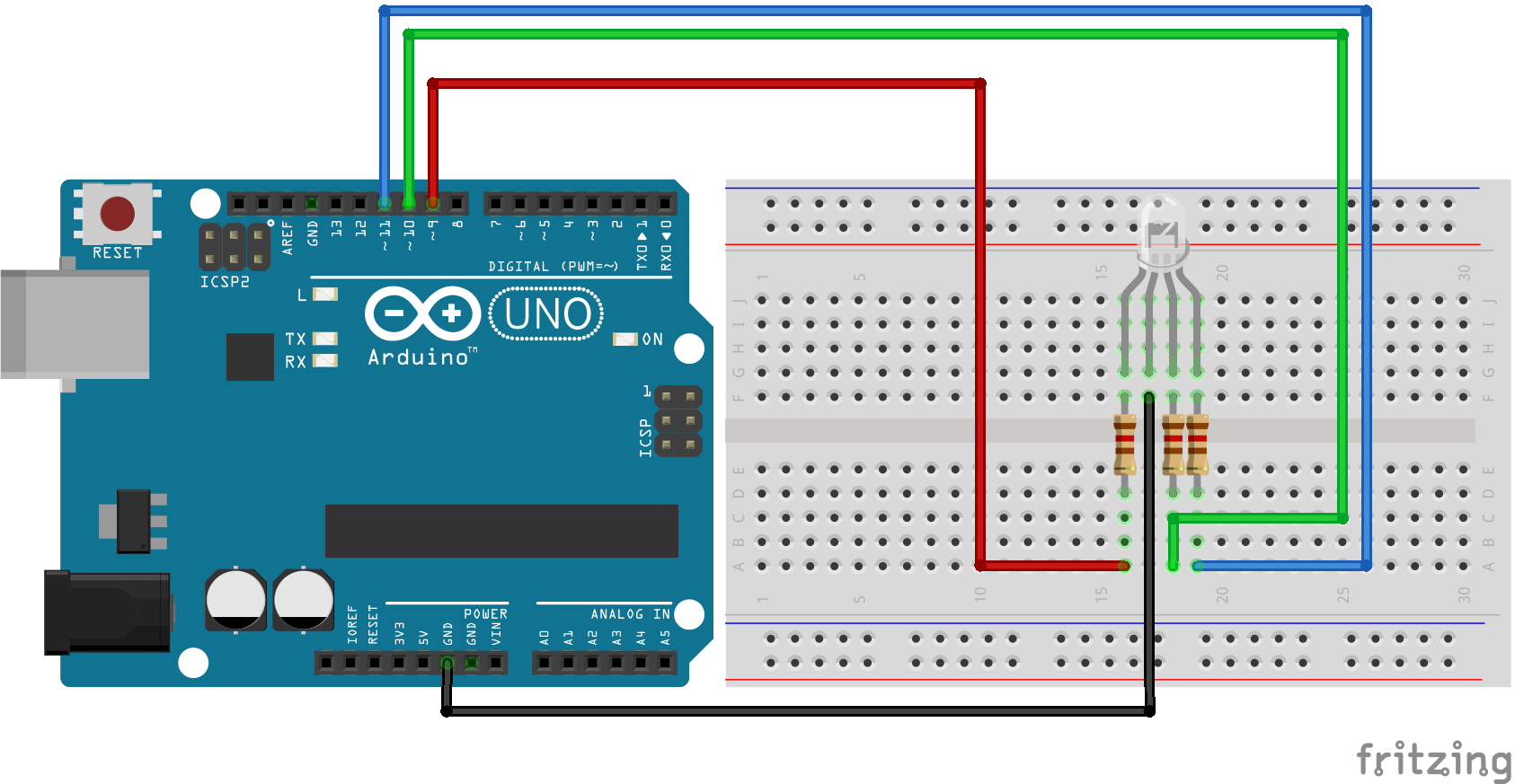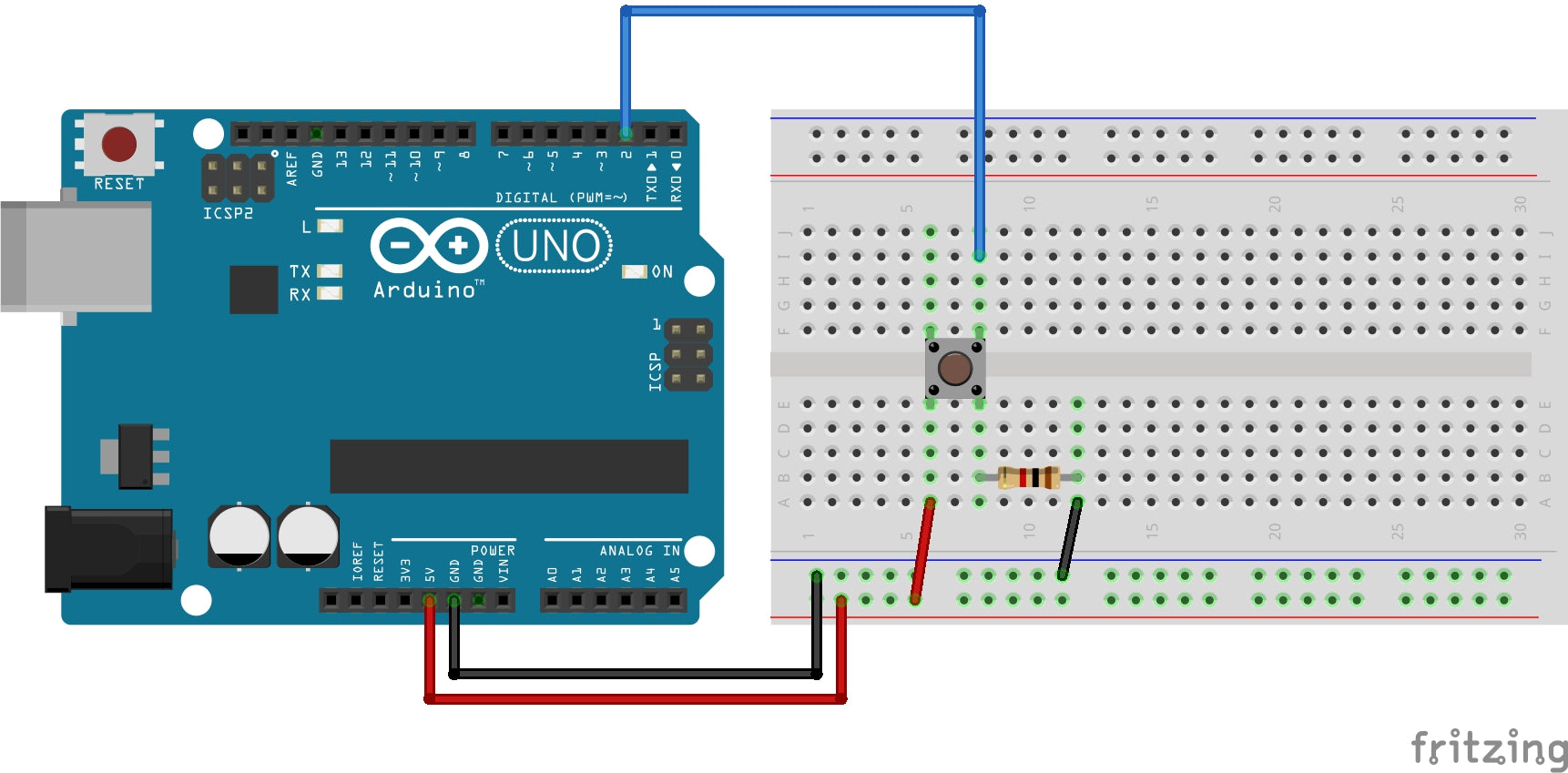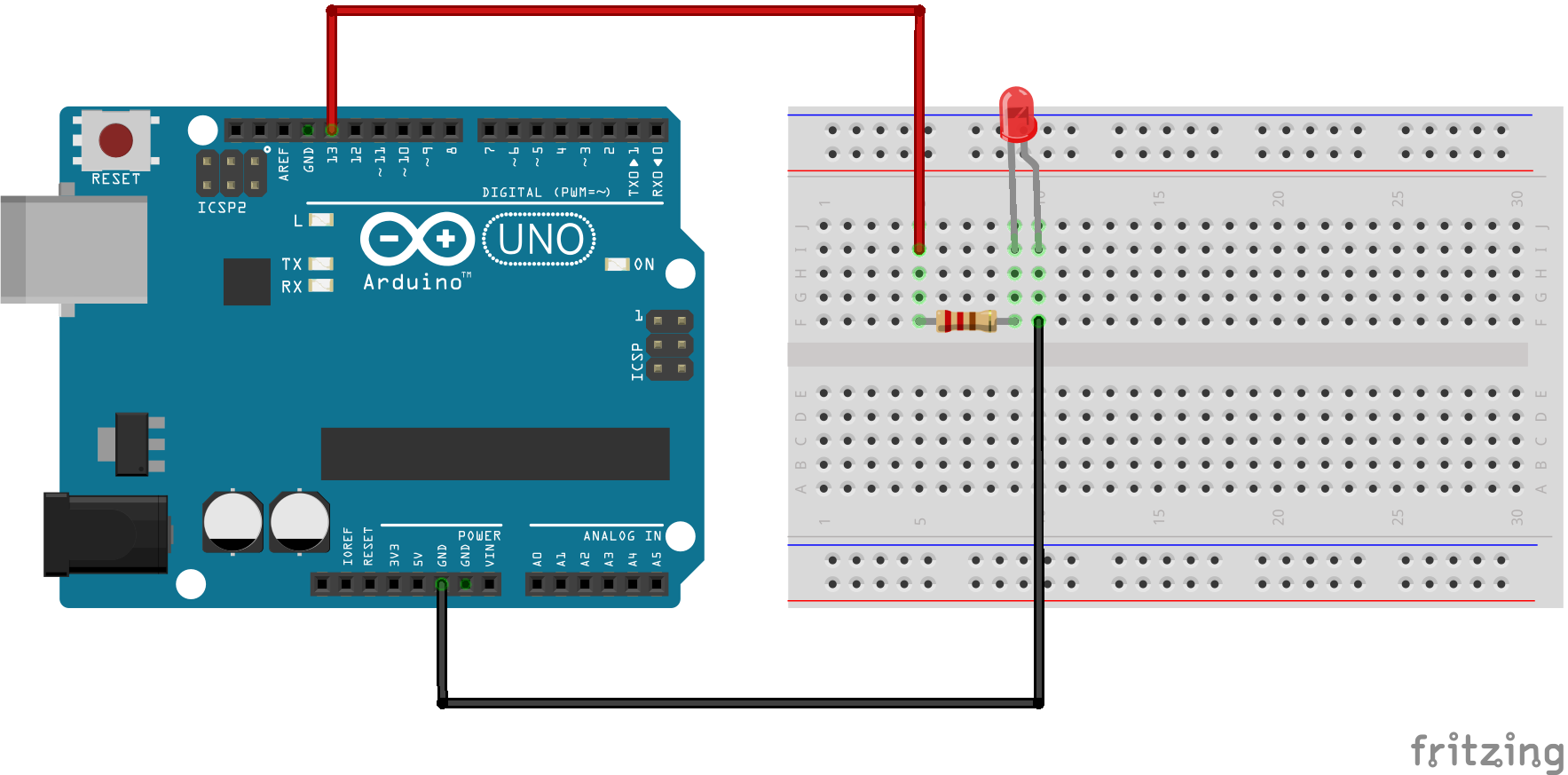# What Is An Electrical Charge?

## 0.0 Introduction

An electron is the smallest particle that exhibits negative electrical charge. When an excess of electrons exists in a material, there is a net negative electrical charge. When a deficiency of electrons exists, there is a net positive electrical charge.

## 1.0 Electrical Charge

The charge of an electron and that of a proton are equal in magnitude. Electrical charge is an electrical property of matter that exists because of an excess or deficiency of electrons. The letter Q symbolizes charge. Static electricity is the presence of a net positive or negative charge in a material.

Materials with charges of opposite polarity are attracted to each other, and materials with charges of the same polarity are repelled. As shown in figure 1, a force acts between charges, as evidenced by the attraction or repulsion. This force is called an electric field.Figure 1. Attraction and repulsion of electrical charges

Coulomb's Law states:

A force (F) exists between two point-source charges ($// $) that is directly proportional to the product of the two charges and inversely proportional to the square of the distance (d) between the charges.

## 2.0 Coulomb: The Unit of Charge

Electrical charge ($// $) is measured in coulombs, symbolized by $// $.

One coulomb is the total charge possessed by $// $electrons. A single electron has a charge of $// $. The total charge $// $, expressed in coulombs, for a given number of electrons is sated in the following formula:

## 3.0 Positive and Negative Charge

Consider a neutral atom -- that is, one that has the same number of electrons and protons and thus has no net charge. As you know, when a valence electron is pulled away from the atom by the application of energy, the atom is left with a net positive charge (more protons than electrons) and becomes a positive ion. If an atom acquires an extra electron in its outer shell, it has a negative charge and becomes a negative ion.

The energy required to free a valence electron is related to the number of electrons in the outer shell. An atom can have up to eight valance electrons. The more complete the outer shell, the more stable the atom and thus the more energy is required to remove an electron.

## 4.0 Check-up

1. What is the symbol for charge?
2. What is the unit of charge, and what is the unit symbol?
3. What causes a positive and negative charge?
4. How much charge, in coulombs, is there in $// $electrons?

•### A Beginner's Guide to Connecting and Programmin...

RGB LEDs are versatile and dynamic lighting solutions that can display a wide range of colors. In this beginner-friendly guide, we'll show you how to connect and program an RGB...

### A Beginner's Guide to Connecting and Programmin...

RGB LEDs are versatile and dynamic lighting solutions that can display a wide range of colors. In this beginner-friendly guide, we'll show you how to connect and program an RGB...

•### Arduino Push Button

This example turns on the built-in LED on pin 13 when you press the button. 1.0 Background Knowledge 1.1 Push Buttons Pushbuttons or switches connect two points in a circuit...

### Arduino Push Button

This example turns on the built-in LED on pin 13 when you press the button. 1.0 Background Knowledge 1.1 Push Buttons Pushbuttons or switches connect two points in a circuit...

•### How to Blink an LED Using Arduino

This project is the "Hello World!" of Arduino. In this project, we are going to learn about physical outputs and two electronic components, while performing the simplest project in the...

### How to Blink an LED Using Arduino

This project is the "Hello World!" of Arduino. In this project, we are going to learn about physical outputs and two electronic components, while performing the simplest project in the...